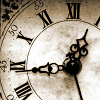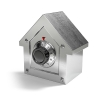# Resources tagged with: Mixed fractions, decimals and percentages

Filter by: Content type:
Age range:
Challenge level:

### There are 4 results

Broad Topics > Fractions, Decimals, Percentages, Ratio and Proportion > Mixed fractions, decimals and percentages### As Easy as 1,2,3

##### Age 11 to 14 Challenge Level:

When I type a sequence of letters my calculator gives the product of all the numbers in the corresponding memories. What numbers should I store so that when I type 'ONE' it returns 1, and when I type. . . .### John's Train Is on Time

##### Age 11 to 14 Challenge Level:

A train leaves on time. After it has gone 8 miles (at 33mph) the driver looks at his watch and sees that the hour hand is exactly over the minute hand. When did the train leave the station?### Mathland Election

##### Age 11 to 14 Challenge Level:

A political commentator summed up an election result. Given that there were just four candidates and that the figures quoted were exact find the number of votes polled for each candidate.### A Change in Code

##### Age 14 to 16 Challenge Level:

There are two sets of numbers. The second is the result of the first after an increase by a constant percentage. How can you find that percentage if one set of numbers is in code?